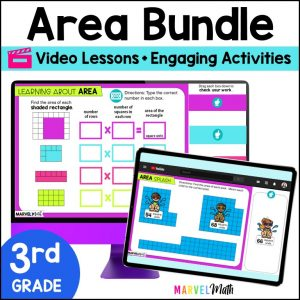Save 10% on your first order with code EXTRA10

# Introduction to Area – Digital Activities + Video Lesson

\$4.00\$16.80
SKU: 5396008 Categories: ,

## Description

The FUN and ENGAGING Introduction to Area Practice Activities and Video Lesson is perfect for your lessons, math stations, review or tutoring. Students will be introduced to Unit Squares to find the Area of Rectangles in these Simple and Easy-to-Use Google Slides. This resource is designed for 2nd and 3rd grade students and aligns to these standards: TEKS 2.9F and CCSS 3.MD.C.5.

What Teachers are saying:

⭐⭐⭐⭐⭐ Most success I’ve every had introducing area. Visual, step by step. Can also be used to reinforce for struggling students in small groups. -Alison L.

⭐⭐⭐⭐⭐ I love that there’s an intro video along with practice slides. I was able to use this for at home learners and in class learners. -Erin C.

The Video Lesson:

• Introduces students to unit squares
• Shows students how to cover a rectangle without gaps or overlaps to find area
• Demonstrates counting unit squares to calculate the area of a rectangle
• Walks students through the Google Slides activities they will complete
• Is approximately 5 minutes
• Student Devices must have access to You-Tube to view the Video Lesson

Topics Include:

• Covering Rectangles with Unit Squares
• Counting Unit Squares to Calculate the Area
• Application of Learning in Real-World Word Problems

What’s Included?

• Video Lesson with Activity Instructions
• 10 Interactive Activities
• Teacher Key in separate Google Slides™ doc that can be assigned to students to check their work at home with their parents
• Directions on how to assign the Google Slides™ activity to students in Google Classroom™

Standards Alignment

CCSS.3.MD.C.5.A A square with side length 1 unit, called “a unit square,” is said to have “one square unit” of area, and can be used to measure area.

CCSS.3.MD.C.5.B A plane figure which can be covered without gaps or overlaps by n unit squares is said to have an area of n square units.

TEKS 2.9F Use concrete models of square units to find the area of a rectangle by covering it with no gaps or overlaps, counting to find the total number of square units, and describing the measurement using a number and the unit.

This is Lesson 1 in a comprehensive bundle that covers all the area topics your students need to learn.

Save 30% on this resource by purchasing the Digital Area Bundle!

Lessons in the Digital Area Bundle Include:

Area Lesson 1 *This Resource*: Understand the Concept of Area by filling Rectangles with Square Units

Area Lesson 2: Find Area by Multiplying the Number of Rows by the number of Unit Squares in Each Row

Area Lesson 3: Multiplying Whole Number Side Lengths to find Area

Area Lesson 4: Area of Irregular Shapes using Unit Squares

Area Lesson 5: Area of Irregular Shapes using Side Lengths

Area Lesson 6: Use Area Models to Prove the Distributive Property

You may also be interested in this coordinating Perimeter Bundle.